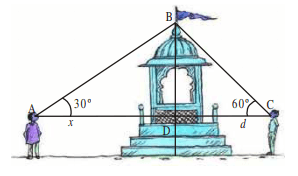# AP Class 10 Maths Chapter 12 Applications of Trigonometry

Trigonometry was first developed for Astronomy and Geography, but now it is used in Engineering, Physics and Chemistry. In the AP Class 10 Maths Chapter 12 Applications of Trigonometry, we learn how to use trigonometric ratios to determine the height and length of an object.

Referring to this AP Board Class 10 Maths Chapter 12 Applications of Trigonometry Notes and Solutions is the best way to master the concepts and prepare for the board exams. Students can see from here how the questions are solved step-wise.

## Using Figures To Solve Problems

We should consider the following, while we solve problems of height and distance.

• Objects such as trees, towers and buildings should be considered linear for mathematical convenience.
• The angle of elevation should be considered with reference to the horizontal line.
• If the height of the observer is not given in the problem, it should be neglected.

Let us look at a few chapter questions in the next section, to better understand.

### Class 10 Maths Chapter 12 Applications of Trigonometry Questions

1. Two men on either side of a temple of 50-meter height look at its top at the angles of elevation 30º and 60º respectively. Find the distance between the two men.Solution:

Consider the distance between the first person and the temple as AD = x and the distance between the second person and temple as CD = d.

$\tan 30^{\circ}=\frac{BD}{AB}$

Substituting the values, we get

$\frac{1}{\sqrt{3}}=\frac{50}{x}$ $x=50\sqrt{3}$……..(1)

From ∆BCD

$\tan 60^{\circ}=\frac{BD}{d}$

Substituting the values, we get

$\sqrt{3}=\frac{50}{d}$ $d=\frac{\sqrt{50}}{3}$…….(2)

From (1) and (2), we can calculate the distance between them as follows,

= x + d

= $x=50\sqrt{3}$ + $d=\frac{\sqrt{50}}{3}$

= $\frac{(50\times 3)+50}{\sqrt{3}}=\frac{200}{\sqrt{3}}$

Simplifying, we get

$\frac{200}{\sqrt{3}}\times \frac{\sqrt{3}}{\sqrt{3}}=66.66\sqrt{3}\,meters$

Stay tuned to BYJU’S to get the latest notification on SSC exams along with AP SSC model papers, exam pattern, marking scheme and more.